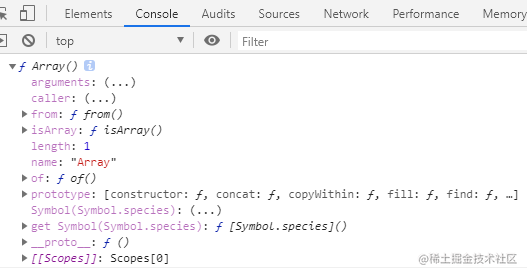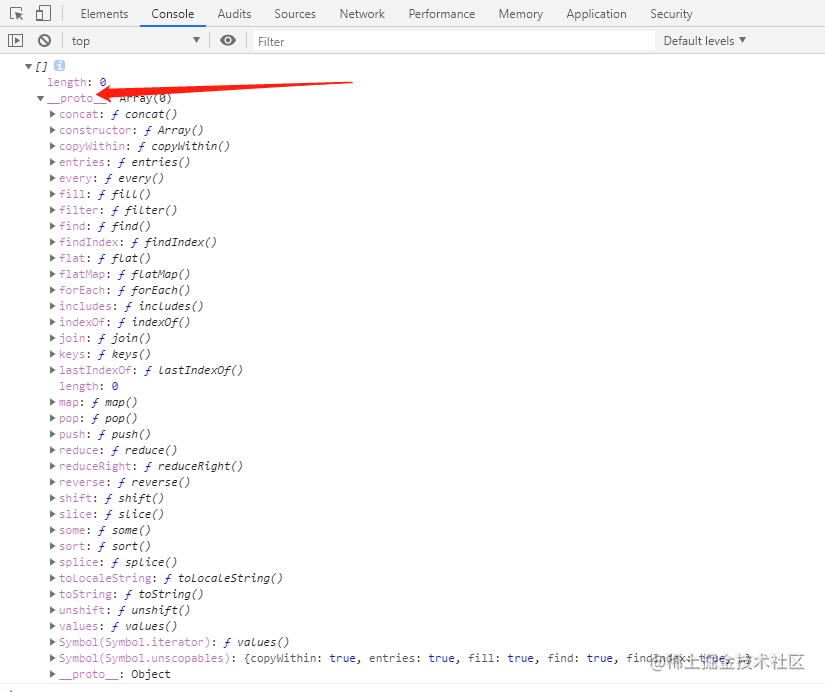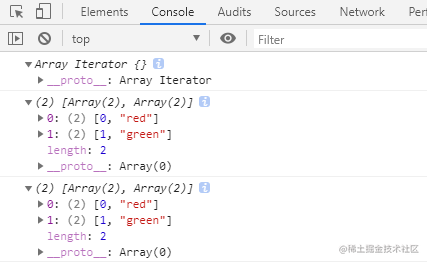# 1.数组介绍

## （2）怎么创建数组？

1. 对象方式创建数组
2. 字面量方式创建数组 创建数组可以通过上面这两种方式，当然也可以通过Array.of()这个函数创建数组。

### 对象方式创建数组

 ``````1 `````` ``````let array = new Array(); ``````

Tips：

 ``````1 `````` ``````let array = Array(); ``````

 ``````1 2 3 4 `````` ``````console.log(new Array(1)); // [empty] console.log(new Array(1,2,3)); // [1, 2, 3] console.log(Array.of(1)); //  console.log(Array.of(1,2,3)); // [1, 2, 3] ``````

Tips：

### 字面量的方式创建数组

 ``````1 `````` ``````let arr = []; ``````

Tips：

# 2.Array对象介绍

## （1）Array介绍

JavaScript的 Array 对象是用于构造数组的全局对象，数组是类似于列表的高阶对象。

Array对象是js内置的用于构造数组的全局对象，数组身上的方法都是继承于Array对象。from() 将类数组结构转换为数组实例 ES6 ×
isArray() 判断数组是否为数组类型 ES6 ×
of() 将一组参数转换为数组实例 ES6 ×

## （2）Array.from()

object 必需，要转换为数组的对象
mapFunction 可选，数组中每个元素要调用的函数
thisValue 可选，映射函数(mapFunction)中的 this 对象

 `````` 1 2 3 4 5 6 7 8 9 10 11 12 13 14 15 16 17 18 19 20 21 22 23 24 25 26 27 28 29 30 31 32 33 34 35 36 37 `````` ``````// 1.类数组对象 let obj = { 1: 'techerM', 2: 15, length: 2 }; console.log(Array.from(obj)); // ["techerM", 15] // 2.字符串 let str = '阿星'; console.log(Array.from(str)); // ["阿", "星"] // 3.集合 let s = new Set().add(1) .add(2) .add(1) .add(4); console.log(Array.from(s)); // [1, 2, 4] // 4.第二个参数，可操作数组中的每个元素 let numbersObj = { 0:1, 1:2, 2:3, 3:2, length: 3 }; console.log(Array.from(numbersObj,item => item*3));//[3, 6, 9] console.log(numbersObj); // {0: 1, 1: 2, 2: 3, 3: 2, length: 3} // 5.第三个参数，指定第二个参数（函数）中this的指向 let numArray = [1,2,3]; var name = '小花'; let res1 = Array.from(numArray,function(item){ return this.name; }) let res2 = Array.from(numArray,function(item){ return this.name; },{name:'小丽'}) console.log(res1); // ["小花", "小花", "小花"] console.log(res2); // ["小丽", "小丽", "小丽"] ``````

Tips：

1.Array.from()是Array对象的私有方法，并不是Array原型prototype下的方法，所以数组继承不到这个方法，数组是没有这个方法的。

2.Array.from()第一个参数类数组对象的条件为：存在length属性，数据为可索引结构，例如下面这个对象就不可进行迭代，因为没有可索引结构

 ``````1 2 3 4 5 `````` ``````let obj = { name: '小丽', value: 100, length: 2 } ``````

3.Array.from()方法不会改变原数组

4.Array.from()中第3个参数设置第二个参数（函数）this的指向，不适用于箭头函数，也就是说如果你第二个参数用的箭头函数的写法，那么你设置第3个参数时将不起作用

### Array.from()方法用于哪些地方

1. 将字符串转换为数组
2. 将集合和映射转换为数组
3. 可以对现有数组进行浅复制
4. 可应用于任何符合条件（length和索引）的可迭代对象

## （3）Array.of()

elementN 任意个参数，将按顺序成为返回数组中的元素。

 ``````1 2 3 4 5 6 7 8 `````` ``````// 一组参数 console.log(Array.of(1,2,3)); //  [1, 2, 3] // 通过 Array.of() 创建数组对象 let arr = Array.of(1); console.log(arr); //  // new Array() 创建数组对象 let arr1 = new Array(1); console.log(arr1); // [empty] ``````

Tips：

## （4）Array.isArray()

obj 必需，要判断的对象。

 ``````1 2 3 4 5 6 `````` ``````let colors = ['red','green']; let name = '小王'; let age = 18; console.log(Array.isArray(colors)); // true console.log(Array.isArray(name)); // false console.log(Array.isArray(age)); // false ``````

# 3.检测数组

Array.isArray() 返回一个布尔类型的值，是数组则返回true，反之false
instanceof 返回一个布尔类型的值，是数组则返回true，反之false
Object.prototype.toString.call() 返回详细的具体类型
 ``````1 2 3 4 5 6 7 8 `````` ``````let array = [1,2]; let obj = {name: '小明'}; console.log(Array.isArray(array)); // true console.log(Array.isArray(obj)); // false console.log(array instanceof Array); // true console.log(obj instanceof Array); // false console.log(Object.prototype.toString.call(array)); // [object Array] console.log(Object.prototype.toString.call(obj)); // [object Object] ``````

Tips：

# 4.数组中的方法

## 数组中的方法总览

 ``````1 `````` ``````console.log([]); ``````push() 向数组的末尾添加一个或多个元素，并返回新的长度
pop() 删除并返回数组的最后一个元素
unshift() 向数组的开头添加一个或更多元素，并返回新的长度
shift() 把数组的第一个元素从其中删除，并返回第一个元素的值
copyWithin() 浅复制数组的一部分到同一数组中的另一个位置，并返回它，不会改变原数组的长度
fill() 向一个已有的数组中插入全部或部分相同的值
reverse() 用于颠倒数组中元素的顺序
sort() 用原地算法对数组的元素进行排序，并返回数组
splice() 向/从数组中添加/删除项目，然后返回被删除的项目
slice() 可提取字符串的某个部分，并以新的字符串返回被提取的部分 ×
concat() 用于合并两个或多个数组。此方法不会更改现有数组，而是返回一个新数组 ×
join() 将一个数组（或一个类数组对象）的所有元素连接成一个字符串并返回这个字符串 ×
every() 对数组中每一项都运行传入的函数，如果对每一项函数都返回true，则这个方法返回true ×
some() 对数组中每一项都运行传入的函数，如果有一项函数返回true，则这个方法返回true ×
filter() 对数组每一项都运行传入的函数，函数返回true的项会组成数组之后返回 ×
map() 对数组每一项都运行传入的函数，返回由每次函数调用的结果构成的数组 ×
forEach() 对数组每一项都运行传入的函数，没有返回值 ×
keys() 返回数组索引的迭代器 ×
values() 返回数组元素的迭代器 ×
entries() 返回 索引/值 对的迭代器 ×
reduce() 对数组中的每个元素执行一个由您提供的reducer函数，将其结果汇总为单个返回值 ×
reduceRight() 从数组的末尾向前将数组中的数组项做累加 ×
indexOf() 返回在数组中可以找到一个给定元素的第一个索引，如果不存在，则返回-1 ×
lastIndexOf() 返回查找元素在数组中的位置，从数组末尾开始向前查找 ×
includes() 用来判断一个数组是否包含一个指定的值，如果包含则返回 true，否则返回false ×
find() 返回数组中满足提供的测试函数的第一个元素的值。否则返回 undefined ×
findIndex() 返回数组中满足提供的测试函数的第一个元素的索引。若没有找到对应元素则返回-1 ×
toSting() 返回由数组中每个值的等效字符串拼接而成的一个用逗号分隔的字符串 ×
toLocaleString() 返回一个逗号分隔的数组值的字符串 ×
constructor() 返回数组函数的引用，也就是调用这个方法的数组本身 ×
flat() 会按照一个可指定的深度递归遍历数组，将所有元素与遍历到的子数组中的元素合并为一个新数组返回 ×
flatMap() 使用映射函数映射每个元素，然后将结果压缩成一个新数组 ×

• 栈和队列方法（4个
• 复制和填充方法（2个
• 排序方法（2个
• 操作方法（4个
• 迭代方法（5个
• 迭代器方法（3个
• 归并方法（2个
• 搜索查找方法（5个
• 转换方法（2个
• 扁平化方法（2个

## （1）栈和队列方法

### push()

push() 向数组的末尾添加一个或多个元素 把指定的值添加到数组后的新长度

newelement1 必需。要添加到数组的第一个元素
newelement2 可选。要添加到数组的第二个元素
newelementX 可选。可添加多个元素
 ``````1 2 3 4 5 6 7 8 `````` ``````let arr = ['js','css','html']; console.log(arr.push('go','node.js')); // 5 console.log(arr); //  ["js", "css", "html", "go", "node.js"] let arr1 = ['js','css','html']; let array = ['go','node.js']; console.log(arr1.push(...array)); // 5 console.log(arr1); // ["js", "css", "html", "go", "node.js"] ``````

 ``````1 2 3 `````` ``````let arr = ['js','css','html']; arr[arr.length] = 'go'; console.log(arr); // ["js", "css", "html", "go"] ``````

### pop()

pop() 删除并返回数组的最后一个元素 返回被删除的元素

 ``````1 2 3 `````` ``````let colors = ['red','green','blue'] console.log(colors.pop()); // blue console.log(colors); // ["red", "green"] ``````

 ``````1 2 3 `````` ``````let colors = ['red','green','blue']; colors.length = colors.length - 1; console.log(colors); //  ["red", "green"] ``````

Tips：

push()和pop()一起使用就构成了后进先出的栈结构

 ``````1 2 3 4 5 `````` ``````let array = []; console.log(array.push('red','green')); // 2 console.log(array); // ["red", "green"] console.log(array.pop()); // green console.log(array); // ["red"] ``````

### unshift()

unshift() 向数组的开头添加一个或更多元素 把指定的值添加到数组后的新长度

newelement1 必需。向数组添加的第一个元素
newelement2 可选。向数组添加的第二个元素
newelementX 可选。可添加若干个元素
 ``````1 2 3 `````` ``````let colors = ['white']; console.log(colors.unshift('red','blue')); // 3 console.log(colors); // ["red", "blue", "white"] ``````

### shift()

shift() 把数组的第一个元素从其中删除 返回第一个元素的值

 ``````1 2 3 `````` ``````let colors = ['red','black']; console.log(colors.shift()); // red console.log(colors); // ["black"] ``````

Tips：

unshift()和shift()一起使用也构成了后进先出的栈结构

unshift()和pop()一起使用构成了先进先出的队列结构

push()和shift()一起使用也构成了先进先出的队列结构

## （2）复制和填充方法

ES6新增了两个方法：批量复制方法 copyWithin(),以及填充数组方法 fill()。使用这两个方法都不会改变数组的大小。同时这两个方法都是包含开始索引，不包含结束索引的。

### copyWithin()

copyWithin() 浅复制数组的一部分到同一数组中的另一个位置 返回修改后的原数组

target 必需。复制到指定目标索引位置
start 可选。元素复制的起始位置
end 可选。停止复制的索引位置 (默认为 array.length)。如果为负值，表示倒数
 ``````1 2 3 4 5 `````` ``````let ints = [0,1,2]; let copyRes = ints.copyWithin(1); console.log(copyRes); //  [0, 0, 1] console.log(ints); //  [0, 0, 1] console.log(copyRes === ints); // true ``````

### fill()

fill() 向一个已有的数组中插入全部或部分相同的值 返回填充后的原数组

value 必需。填充的值
start 可选。开始填充位置
end 可选。停止填充位置 (默认为 array.length)
 ``````1 2 3 4 5 6 7 8 `````` ``````let zeroes = [0,0,0,0,0]; let zeroesRes = zeroes.fill(5); console.log(zeroes); // [5, 5, 5, 5, 5] console.log(zeroesRes === zeroes); // true console.log(zeroes.fill(3,1)); // [5, 3, 3, 3, 3] console.log(zeroes); // [5, 3, 3, 3, 3] console.log(zeroes.fill(1,3,5)); // [5, 3, 3, 1, 1] console.log(zeroes); // [5, 3, 3, 1, 1] ``````

Tips：

copyWithin()和fill()会忽略超出数组边界，零长度以及方向相反的索引范围

 `````` 1 2 3 4 5 6 7 8 9 10 11 12 13 `````` ``````let ints = [0,1,2,3]; // 索引超出边界，忽略 ints.copyWithin(1,10,13); console.log(ints); // [0,1,2,3] // 索引过低，超出边界，忽略 ints.copyWithin(2,-15,-12); console.log(ints); // [0,1,2,3] // 索引反向，忽略 ints.copyWithin(1,3,1); console.log(ints); // [0,1,2,3] // 部分索引可用，填充可用索引部分 ints.copyWithin(1,2,6); console.log(ints); // [0,2,3,3] ``````
 `````` 1 2 3 4 5 6 7 8 9 10 11 12 13 14 15 16 17 `````` ``````let zeroes = [0,0,0,0,0]; // 当开始索引和结束索引取负值时，相当于：将开始索引和结束索引都加上 zeroes.length // zeroes.fill(8,1,4); zeroes.fill(8,-4,-1); console.log(zeroes); // [0,8,8,8,0] // 索引超出边界，忽略 zeroes.fill(1,10,15); console.log(zeroes); // [0,8,8,8,0] // 索引过低，超出边界，忽略 zeroes.fill(1,-15,-12); console.log(zeroes); // [0,8,8,8,0] // 索引反向，忽略 zeroes.fill(1,3,1); console.log(zeroes); // [0,8,8,8,0] // 部分索引可用，填充可用索引部分 zeroes.fill(1,3,10); console.log(zeroes); // [0, 8, 8, 1, 1] ``````

## （3）排序方法

### reverse()

reverse() 用于颠倒数组中元素的顺序 返回颠倒后的原数组

 ``````1 2 3 4 `````` ``````let value = [1,2,3,4,5,6]; console.log(value.reverse()); // [6, 5, 4, 3, 2, 1] console.log(value); // [6, 5, 4, 3, 2, 1] console.log(value.reverse() === value); // true ``````

### sort()

sort() 用原地算法对数组的元素进行排序 返回排序后的原数组

sortby 可选。规定排序顺序。必须是函数
• 当不传参数时

 ``````1 2 `````` ``````let value = [1,2,3,5,8,9,10,16,20,50]; console.log(value.sort()); //  [1, 10, 16, 2, 20, 3, 5, 50, 8, 9] ``````
• 传入比较函数

 ``````1 2 3 4 5 6 7 8 `````` ``````let value = [1,2,3,5,8,9,10,16,20,50]; function compare(a,b) { if(a < b) return -1 else if(a > b) return 1 else return 0; } value.sort(compare); console.log(value); // [1, 2, 3, 5, 8, 9, 10, 16, 20, 50] ``````

 ``````1 2 3 4 5 6 7 8 `````` ``````let value = [1,2,3,5,8,9,10,16,20,50]; function compare(a,b) { if(a > b) return -1 else if(a < b) return 1 else return 0; } value.sort(compare); console.log(value); // [50, 20, 16, 10, 9, 8, 5, 3, 2, 1] ``````

 ``````1 2 3 `````` ``````let value = [1,2,3,5,8,9,10,16,20,50]; value.sort((a,b) => a > b ? -1 : a > b ? 1 : 0); console.log(value); // [50, 20, 16, 10, 9, 8, 5, 3, 2, 1] ``````

 ``````1 2 3 `````` ``````let value = [1,2,3,5,8,9,10,16,20,50]; value.sort((a,b) => b - a); // a - b 升序，b - a 降序 console.log(value); // [50, 20, 16, 10, 9, 8, 5, 3, 2, 1] ``````
• 数组对象按某个属性排序
 `````` 1 2 3 4 5 6 7 8 9 10 `````` ``````let array = [     {'name':'js','grade':80},     {'name':'java','grade':50},     {'name':'go','grade':100}, ] array.sort(function(a,b) {     return b.grade - a.grade; }) console.log(array); // [{'name':'go','grade':100},{'name':'js','grade':80},{'name':'java','grade':50}] ``````
• 手写一个sort函数
 `````` 1 2 3 4 5 6 7 8 9 10 11 12 13 14 15 16 17 `````` ``````function sort(array,callback) { for (const n in array) { for (const m in array) { if(callback(array[n],array[m]) < 0){ const temp = array[n]; array[n] = array[m]; array[m] = temp; } } } return array; }; let arr = [10,52,2,3,7]; sort(arr,function(a,b){ return a - b }) console.log(arr); // [2, 3, 7, 10, 52] ``````

Tips：

## （4）操作方法

### splice()

splice() 向/从数组中添加/删除项目 返回被删除元素的组成的数组

index 必需。整数，规定添加/删除项目的位置，使用负数可从数组结尾处规定位置
howmany 必需。要删除的项目数量。如果设置为 0，则不会删除项目
index 可选。向数组添加的新项目
 ``````1 2 3 4 5 6 `````` ``````let nums = [1,2,3,4,5,6,7,8,9,10]; console.log(nums.splice(0,2)); // [1, 2] console.log(nums); // [3, 4, 5, 6, 7, 8, 9, 10] let colors = ['red','green']; console.log(colors.splice(0,1,'black','white')); // ["red"] console.log(colors); // ["black", "white", "green"] ``````

Tips：

 ``````1 2 3 4 5 `````` ``````let nums = [1,2,3,4,5,6,7,8,9,10]; console.log(nums.splice()); // [] console.log(nums); // [1,2,3,4,5,6,7,8,9,10] console.log(nums.splice(3)); // [4, 5, 6, 7, 8, 9, 10] console.log(nums); // [1, 2, 3] ``````

### slice()

slice() 可提取字符串的某个部分 以新数组的形式返回被提取的部分 ×

start 可选。规定从何处开始选取，包含这个位置
end 可选。规定从何处结束选取，不包含这个位置
 ``````1 2 3 4 5 6 7 8 `````` ``````let colors = ['red','blue']; let colors2 = colors.slice(); let colors3 = colors.slice(1); let colors4 = colors.slice(0,1); console.log(colors); //  ["red", "blue"] console.log(colors2); // ["red", "blue"] console.log(colors3); // ["blue"] console.log(colors4); // ["red"] ``````

Tips：

 `````` 1 2 3 4 5 6 7 8 9 10 11 12 13 14 15 16 17 18 19 20 `````` `````` let colors = ['red','blue','green']; // 这个数组的长度为3，所以colors.slice(-3,-1) 相当于 colors.slice(0,2) console.log(colors.slice(-3,-1)); //  ["red", "blue"] console.log(colors.slice(0,2)); //  ["red", "blue"] // 因为colors.slice(-6,-4) 加上数组长度分别为 -3，-1，所以返回空值 // 但是colors.slice(-6,-4) 结果并不等于 colors.slice(-3,-1)的结果 // 因为 colors.slice(-3,-1) 相当于 colors.slice(0,2) console.log(colors.slice(-6,-4)); // [] console.log(colors.slice(-3,-1)); // ["red", "blue"] // colors.slice(-8,-1) 加上数组长度分别为 -5，2，有一部分是在数组索引范围内的 // 所以这部分是生效的 console.log(colors.slice(-8,-1)); // ["red", "blue"] // colors.slice(-5,2) 加上数组长度为 -2，2 // 但是 colors.slice(-2,2) 加上数组长度为 1，2 // 所以他们结果是不同的 console.log(colors.slice(-5,2)); // ["red", "blue"] console.log(colors.slice(-2,2)); // ["blue"] ``````

### concat()

concat() 用于合并两个或多个数组 返回合并后的新创建的数组 ×

arrayX 必需。该参数可以是具体的值，也可以是数组对象。可以是任意多个

concat不仅可以合并数组，也可以将字符串合并到数组中去

 ``````1 2 3 4 5 `````` ``````let colors = ['red','green']; let newColors = ['brown','blue']; console.log(colors.concat(newColors)); // ["red", "green", "brown", "blue"] console.log(colors.concat(['yellow'],newColors)); // ["red", "green", "yellow", "brown", "blue"] console.log(colors.concat('white',newColors)); // ["red", "green", "white", "brown", "blue"] ``````

Tips：

concat() 将字符串合并到数组中有点类似于push() ，但是concat() 不会改变原数组，而push() 会改变原数组，同时，concat() 的返回值是合并后的新数组，而push() 的返回值是添加元素后的原数组的长度

 ``````1 2 3 4 5 6 7 `````` ``````let colors = ['red']; console.log(colors.concat('green','blue')); // ["red", "green", "blue"] console.log(colors); // ["red"] let newColors = ['red']; console.log(colors.push('green','blue')); // 3 console.log(colors); // ["red", "green", "blue"] ``````

### join()

join() 将一个数组的所有元素连接成一个字符串并返回 返回连接后的字符串 ×

separator 可选。指定要使用的分隔符。如果省略该参数，则使用逗号作为分隔符
 ``````1 2 3 4 5 6 `````` ``````let colors = ['red','green','blue']; console.log(colors.join()); // red,green,blue console.log(colors.join(',')); // red,green,blue console.log(colors.join('||')); // red||green||blue console.log(colors.join(undefined)); // red,green,blue console.log(colors.join(null)); // rednullgreennullblue ``````

Tips：

 ``````1 2 3 4 `````` ``````let colors = ['red','green','blue']; console.log(colors.join(undefined)); // red,green,blue let colors1 = ['red',null,undefined,'blue']; console.log(colors1.join()); // red,,,blue ``````

## （5）迭代方法

JavaScript 为数组定义了5个迭代方法。

### every()

every() 对数组中每一项都运行传入的函数，如果对 每一项 函数都返回 true ，则这个方法返回 true 返回一个布尔类型的值 ×

function(currentValue, index,arr) 必须。函数，数组中的每个元素都会执行这个函数
thisValue 可选。对象作为该执行回调时使用，传递给函数，用作 “this” 的值

currentValue 必须。当前元素的值
index 可选。当前元素的索引值
arr 可选。当前元素属于的数组对象
 ``````1 2 3 `````` ``````let numbers = [1,2,3,4,5,4,3,2,1]; let everyResult = numbers.every((item,index,numbers) => item > 2); console.log(everyResult); // false ``````

Tips：

 ``````1 2 3 `````` ``````let numbers = []; let everyResult = numbers.every((item,index,numbers) => item > 5); console.log(everyResult); // true ``````

### some()

some() 对数组中每一项都运行传入的函数，如果 有一项 函数返回 true ，则这个方法返回 true 返回一个布尔类型的值 ×

function(currentValue, index,arr) 必须。函数，数组中的每个元素都会执行这个函数
thisValue 可选。对象作为该执行回调时使用，传递给函数，用作 “this” 的值

currentValue 必须。当前元素的值
index 可选。当前元素的索引值
arr 可选。当前元素属于的数组对象

 ``````1 2 3 4 5 `````` ``````let numbers = [1,2,3,4,5,4,3,2,1]; let everyResult = numbers.every((item,index,numbers) => item > 2); console.log(everyResult); // false let someResult = numbers.some((item,index,numbers) => item > 2); console.log(someResult); // true ``````

### filter()

filter() 对数组每一项都运行传入的函数，函数返回 true 的项会组成数组之后返回 返回一个数组 ×

function(currentValue, index,arr) 必须。函数，数组中的每个元素都会执行这个函数
thisValue 可选。对象作为该执行回调时使用，传递给函数，用作 “this” 的值

currentValue 必须。当前元素的值
index 可选。当前元素的索引值
arr 可选。当前元素属于的数组对象
 ``````1 2 3 `````` ``````let numbers = [1,2,3,4,5,4,3,2,1]; let filterResult = numbers.filter((item,index,numbers) => item > 2); console.log(filterResult); // [3, 4, 5, 4, 3] ``````

### map()

map() 对数组每一项都运行传入的函数，返回由每次函数调用的结果构成的数组 返回一个数组 ×

function(currentValue, index,arr) 必须。函数，数组中的每个元素都会执行这个函数
thisValue 可选。对象作为该执行回调时使用，传递给函数，用作 “this” 的值

currentValue 必须。当前元素的值
index 可选。当前元素的索引值
arr 可选。当前元素属于的数组对象
 ``````1 2 3 `````` ``````let numbers = [1,2,3,4,5,4,3,2,1]; let mapResult = numbers.map((item,index,number) => item*2); console.log(mapResult); //  [2, 4, 6, 8, 10, 8, 6, 4, 2] ``````

Tips：

 `````` 1 2 3 4 5 6 7 8 9 10 `````` ``````let numbers = [1,2,3,4,5,4,3,2,1]; let filterResult = numbers.filter((item,index,number) => item * 2); let mapResult = numbers.map((item,index,number) => item * 2); let filterResult1 = numbers.filter((item,index,number) => item > 2); let mapResult1 = numbers.map((item,index,number) => item > 2); console.log(filterResult); //  [1, 2, 3, 4, 5, 4, 3, 2, 1] console.log(mapResult); // [2, 4, 6, 8, 10, 8, 6, 4, 2] console.log(filterResult1); //  [3, 4, 5, 4, 3] console.log(mapResult1); //  [false, false, true, true, true, true, true, false, false] console.log(numbers); //  [1, 2, 3, 4, 5, 4, 3, 2, 1] ``````

### forEach()

forEach() 对数组每一项都运行传入的函数，没有返回值 undefined ×

function(currentValue, index,arr) 必须。函数，数组中的每个元素都会执行这个函数
thisValue 可选。对象作为该执行回调时使用，传递给函数，用作 “this” 的值

currentValue 必须。当前元素的值
index 可选。当前元素的索引值
arr 可选。当前元素属于的数组对象
 ``````1 2 3 4 5 6 `````` ``````let numbers = [1,2,3,4,5,4,3,2,1]; let forEachResult = numbers.forEach((item,index,numbers) => { return item*5 }); console.log(forEachResult); // undefined console.log(numbers); // [1, 2, 3, 4, 5, 4, 3, 2, 1] ``````

forEach()本质上就是循环遍历数组，没有返回值，也不会改变原数组。

## （6）迭代器方法

ES6 中，Array 的原型上暴露了 3 个用于检索数组内容的方法，keys()values()entries()

### keys()

keys() 返回数组索引的迭代器 返回一个类数组对象 ×

obj 可选。要返回其枚举自身属性的对象
 ``````1 2 3 4 `````` ``````let colors = ['red','green']; console.log(colors.keys()); // Array Iterator {} console.log(Array.from(colors.keys())); // [0, 1] console.log(Array.of(...colors.keys())); // [0, 1] ``````

### values()

values() 返回数组元素的迭代器 返回一个类数组对象 ×

obj 可选。被返回可枚举属性值的对象
 ``````1 2 3 4 `````` ``````let colors = ['red','green']; console.log(colors.values()); // Array Iterator {} console.log(Array.from(colors.values())); //  ["red", "green"] console.log(Array.of(...colors.values())); //  ["red", "green"] ``````

### entries()

entries() 返回 索引/值 对的迭代器 返回一个类数组对象 ×

obj 可选。被返回可枚举属性值的对象
 ``````1 2 3 4 `````` ``````let colors = ['red','green']; console.log(colors.entries(colors)); // Array Iterator {} console.log(Array.from(colors.entries())); // [Array(2), Array(2)] console.log(Array.of(...colors.entries())); // [Array(2), Array(2)] ``````Tips：

## （7）归并方法

JavaScript 为数组提供了两个归并方法：reduce()reduceRight()

### reduce()

reduce() 对数组中的每个元素执行一个由您提供的reducer函数 函数处理的结果，可以是任何类型 ×

function(currentValue, index,arr) 必需。用于执行每个数组元素的函数
initialValue 可选。传递给函数的初始值

total 必需。初始值, 或者计算结束后的返回值
currentValue 必需。当前元素
currentIndex 可选。当前元素的索引
arr 可选。当前元素所属的数组对象
 ``````1 2 3 4 `````` ``````let numbers = [1,2,3,2,1]; let sum = numbers.reduce((prev,cur,index,array) => prev + cur); console.log(sum); // 9 console.log(numbers); //  [1, 2, 3, 2, 1] ``````

### reduceRight()

reduceRight() 从数组的末尾向前将数组中的数组项做累加 函数处理的结果，可以是任何类型 ×

function(currentValue, index,arr) 必需。用于执行每个数组元素的函数
initialValue 可选。传递给函数的初始值

total 必需。初始值, 或者计算结束后的返回值
currentValue 必需。当前元素
currentIndex 可选。当前元素的索引
arr 可选。当前元素所属的数组对象
 ``````1 2 3 4 `````` ``````let numbers = [1,2,3,4,5]; let sum = numbers.reduceRight((prev,cur,index,array) => prev + cur); console.log(sum); // 15 console.log(numbers); // [1, 2, 3, 4, 5] ``````

Tips：

## （8）搜索查找方法

JavaScript 提供两类搜索数组的方法：按严格相等搜索和按断言函数搜索。

JavaScript 提供了 3 个严格相等的搜索方法：indexOf()lastIndexOf()includes()

### indexOf()

indexOf() 返回在数组中可以找到一个给定元素的第一个索引 返回数值类型 ×

searchvalue 必需。规定需检索的字符串值
fromindex 可选的整数参数。规定在字符串中开始检索的位置（包含这个位置）
 ``````1 2 3 4 5 `````` ``````let numbers = [1,2,3,4,5]; console.log(numbers.indexOf(4)); // 3 console.log(numbers.indexOf(4,3)); // 3 // 如果没找到就返回 -1 console.log(numbers.indexOf(40)); // -1 ``````

### lastIndexOf()

lastIndexOf() 返回查找元素在数组中的位置，从数组末尾开始向前查找 返回数值类型 ×

searchvalue 必需。规定需检索的字符串值
fromindex 可选的整数参数。规定在字符串中开始检索的位置（包含这个位置）
 ``````1 2 3 4 5 `````` ``````let numbers = [1,2,3,4,5,4,3,2,1]; console.log(numbers.lastIndexOf(4)); // 5 console.log(numbers.lastIndexOf(4,4)); // 3 // 如果没找到就返回 -1 console.log(numbers.lastIndexOf(40)); // -1 ``````

### includes()

includes() 用来判断一个数组是否包含一个指定的值 返回布尔类型 ×

searchvalue 必需。规定需检索的字符串值
fromindex 可选的整数参数。规定在字符串中开始检索的位置（包含这个位置）
 ``````1 2 3 4 `````` ``````let numbers = [1,2,3,4,5,4,3,2,1]; console.log(numbers.includes(4)); // true console.log(numbers.includes(4,7)); // false console.log(numbers.includes(40)); // false ``````

JavaScript 也允许按照定义的断言函数搜索数组，find()findIndex() 使用了断言函数。

### find()

find() 返回数组中满足提供的测试函数的第一个元素的值 返回符合条件的元素值，可以是任何类型 ×

function(currentValue, index,arr) 必须。函数，数组中的每个元素都会执行这个函数
thisValue 可选。对象作为该执行回调时使用，传递给函数，用作 “this” 的值

currentValue 必须。当前元素的值
index 可选。当前元素的索引值
arr 可选。当前元素属于的数组对象
 `````` 1 2 3 4 5 6 7 8 9 10 11 `````` ``````const people = [ { name: 'Matt', age: 27 }, { name: 'xiaoming', age: 29 } ]; console.log(people.find((element, index, array) => element.age > 28)); // {name: "xiaoming", age: 29} ``````

### findIndex()

findIndex() 返回数组中满足提供的测试函数的第一个元素的索引 返回一个数值类型的值 ×

function(currentValue, index,arr) 必须。函数，数组中的每个元素都会执行这个函数
thisValue 可选。对象作为该执行回调时使用，传递给函数，用作 “this” 的值

currentValue 必须。当前元素的值
index 可选。当前元素的索引值
arr 可选。当前元素属于的数组对象
 `````` 1 2 3 4 5 6 7 8 9 10 11 `````` ``````const people = [ { name: 'Matt', age: 27 }, { name: 'xiaoming', age: 29 } ]; console.log(people.findIndex((element, index, array) => element.age > 28)); // 1 ``````

## （9）转换方法

JavaScript 中所有对象都有 toSting()toLocaleString()valueOf() 方法，其中，valueOf() 返回的是数组本身。

### toSting()

toSting() 返回由数组中每个值的等效字符串拼接而成的一个用逗号分隔的字符串 返回字符串类型 ×

 ``````1 2 3 `````` ``````let numbers = [1,2,3,4,5]; let stringNumbers = numbers.toString(); console.log(stringNumbers); // 1,2,3,4,5 ``````

### toLocaleString()

toLocaleString() 返回一个逗号分隔的数组值的字符串 返回字符串类型 ×

 ``````1 2 3 `````` ``````let numbers = ; let stringNumbers = numbers.toLocaleString(); console.log(stringNumbers); // 15,000,000 ``````

## （10）扁平化方法

JavaScript 中提供了两个数组扁平化的方法：flat()flatMap()

### flat()

flat() 会按照一个可指定的深度递归遍历数组，将所有元素与遍历到的子数组中的元素合并为一个新数组返回 返回一个数组类型 ×

depth 可选。指定要提取嵌套数组的结构深度，默认值为 1
 ``````1 2 3 4 `````` ``````let array = [1,5,9,8,,[2,[1,[1,3,[1,9]]]]]; console.log(array.flat(2));// [1, 5, 9, 8, 1, 2, 1, Array(3)] //使用 Infinity，可展开任意深度的嵌套数组 console.log(array.flat(Infinity)); //  [1, 5, 9, 8, 1, 2, 1, 1, 3, 1, 9] ``````

### flatMap()

flatMap() 使用映射函数映射每个元素，然后将结果压缩成一个新数组 返回一个数组类型 ×

function(currentValue, index,arr) 必须。函数，数组中的每个元素都会执行这个函数
thisValue 可选。对象作为该执行回调时使用，传递给函数，用作 “this” 的值

currentValue 必须。当前元素的值
index 可选。当前元素的索引值
arr 可选。当前元素属于的数组对象
 ``````1 2 3 `````` ``````let array = [1,5,9,8]; let flatMapRes = array.flatMap((item,index,array) => item * 3); console.log(flatMapRes); // [3, 15, 27, 24] ``````

# 5.总结